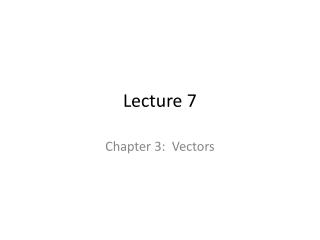DownloadDownload PresentationLecture 7

# Lecture 7

Télécharger la présentation## Lecture 7

- - - - - - - - - - - - - - - - - - - - - - - - - - - E N D - - - - - - - - - - - - - - - - - - - - - - - - - - -
##### Presentation Transcript

1. Lecture 7 Chapter 3: Vectors

2. Chapter 3 Vectors and Motion in Two Dimensions Topics: • Vectors, coordinate systems, and components • Projectile motion • Circular motion Sample question: The archer fish shoots a stream of water droplets at an insect. What determines the path that the droplets take through the air? Slide 3-1

3. Looking Back: What You Already Know • From this class: • In Chapter 1 you learned what a vector is and learned how to add vectors. • In Chapter 2 you learned about motion in one dimension. Motion in two dimensions will build naturally on this foundation. • From previous classes: • You probably know that any two objects in free fall will undergo similar motions; if you drop two objects from the same height at the same time, they will hit the ground simultaneously. • You might well have learned a bit about vectors in another course. Slide 3-2

4. The acceleration of a particle in projectile motion 1.points along the path of the particle. 2. Is directed horizontally 3. vanishes at the particle’s highest point. 4. is directed down at all times. 5. Is zero.

5. Vectors Slide 3-9

6. Vector addition—graphical method A C = A + B B

7. Vector subtraction—graphical method A -B D= A -B -B A B

8. Representing a vector (2-dimensions) Need magnitude (length) and direction y A θ x Length = |A| = magnitude of A

9. Multiplication by a scalar What is a scalar? A number B = cA y B = 3A A x

10. Special Cases • Multiply by c = -1, reverses direction of vector • Multiply by c = 0, result is the zero vector

11. Checking Understanding An arrow is launched vertically upward. It moves straight up to a maximum height, then falls to the ground. The trajectory of the arrow is noted. Which graph best represents the vertical velocity of the arrow as a function of time? Ignore air resistance; the only force acting is gravity. Slide 2-28

12. Answer An arrow is launched vertically upward. It moves straight up to a maximum height, then falls to the ground. The trajectory of the arrow is noted. Which graph best represents the vertical velocity of the arrow as a function of time? Ignore air resistance; the only force acting is gravity. Slide 2-29

13. The figure below shows five arrows with differing masses that were launched straight up with the noted speeds. Rank the arrows, from greatest to least, on the basis of the maximum height the arrows reach. Ignore air resistance; the only force acting is gravity. Slide 2-30

14. Problem • Spud Webb, height 5'7", was one of the shortest basketball players to play in the NBA. But he had an impressive vertical leap; he was reputedly able to jump 110 cm off the ground. To jump this high, with what speed would he leave the ground?

15. Problem • A football is punted straight up into the air; it hits the ground 5.2 s later. What was the greatest height reached by the ball? With what speed did it leave the kicker’s foot?

16. Problem • Passengers on The Giant Drop, a free-fall ride at Six Flags Great America, sit in cars that are raised to the top of a tower. The cars are then released for 2.6 s of free fall. How fast are the passengers moving at the end of this speeding up phase of the ride? If the cars in which they ride then come to rest in a time of 1.0 s, what is acceleration (magnitude and direction) of this slowing down phase of the ride? Given these numbers, what is the minimum possible height of the tower?

17. Additional Clicker Questions • Mike jumps out of a tree and lands on a trampoline. The trampoline sags 2 feet before launching Mike back into the air. At the very bottom, where the sag is the greatest, Mike’s acceleration is: • Upward • Downward • Zero Slide 2-36

18. Homework Friday • Reading: 3: 2-3 Vector components • Problems: 2: 23, 28, 29, 31, 33, 48, 52; 3: CQ1, 1